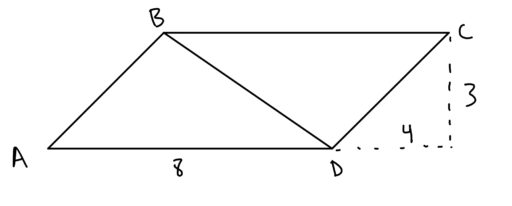Rectangles: four sides connected by four 90-degree angles

Rectangle Area Formula: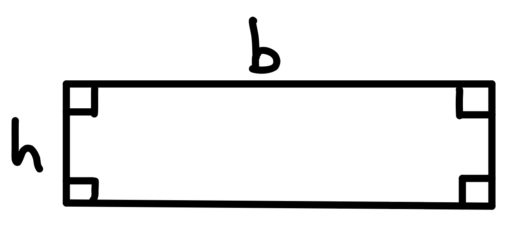Squares: four sides of equal length connected by four 90-degree angles.

Square Area Formula: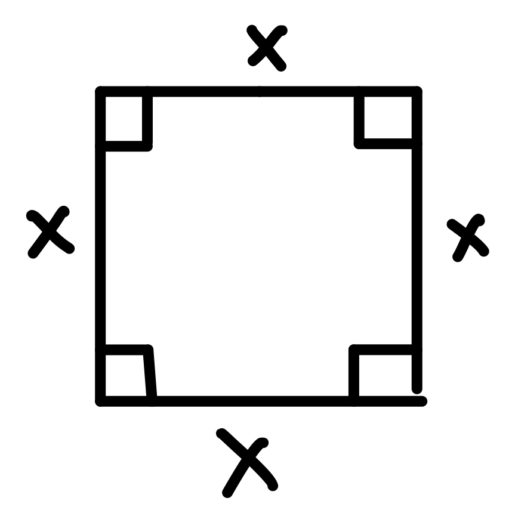Parallelograms: four-sided figure where opposite sides are parallel

Parallelogram Area Formula: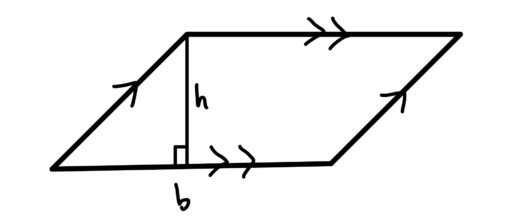Parallelograms also have the nice property that opposite angles are of the same degree, and opposite sides are the same length.

Rhombus: a parallelogram with equal sides

Rhombus Area Formula: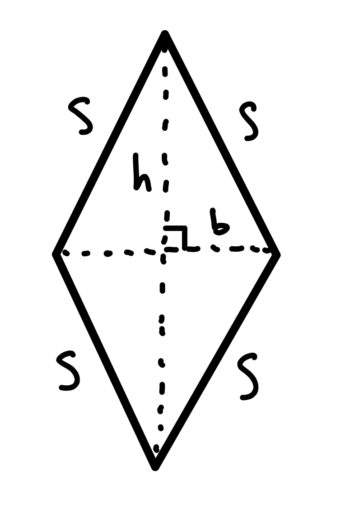Trapezoids: four-sided figure with one pair of parallel opposing sides

Trapezoid Area Formula: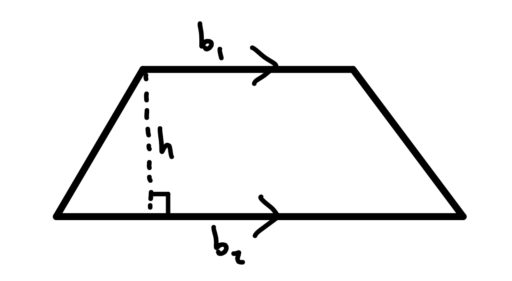Now a lot of these definitions overlap with others, so here is a diagram that explains how all of these shapes relate to one another: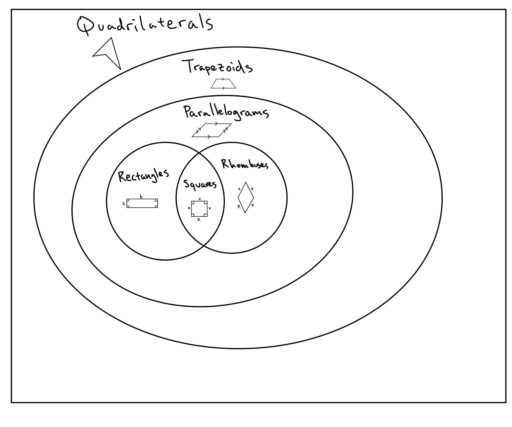Finally, one fact that is sometimes helpful in solving problems with quadrilaterals is:

Quadrilaterals Have 360 Degrees: The sum of the angles in a quadrilateral is 360.

This fact will come up again when we talk about finding the values of unknown angles.

Practice Problems:

1. Find the area of the entire figure below.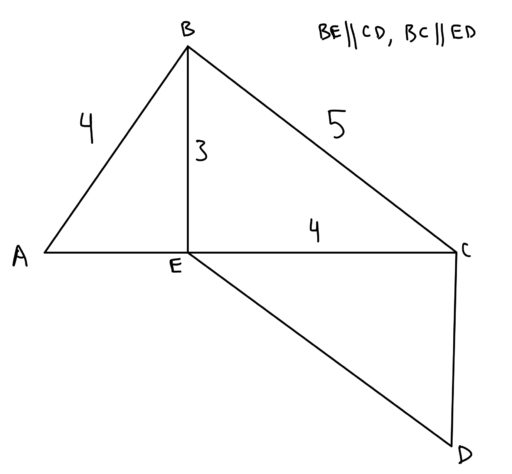2. Find the area of the entire figure below.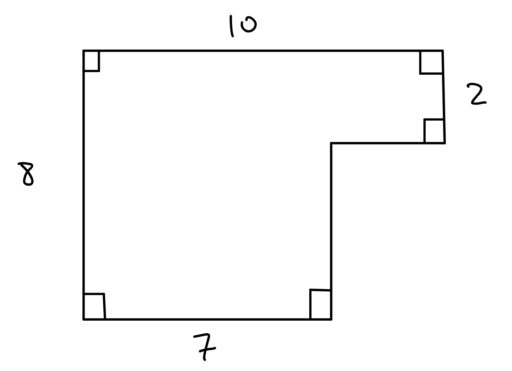3. The area of the below parallelogram is 30,is perpendicular to, and the ratio of the area ofto the area ofis 1 to 3. What is the value of?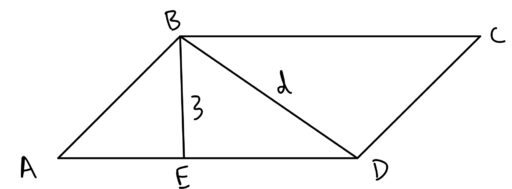4. The dotted lines below meet at a right angle. Find